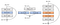# Reading: SqueezeNext — Hardware-Aware Neural Network Design (Image Classification)

## Outperforms AlexNet, VGGNet, SqueezeNet, MobileNetV1 With Lower Complexity or Less inference Time

In this story, SqueezeNext: Hardware-Aware Neural Network Design, by UC Berkeley, is briefly presented. This network, SqueezeNext:

• matches AlexNet’s accuracy on the ImageNet benchmark with 112× fewer parameters.
• achieves VGG-19 accuracy with only 4.4 Million parameters, 31× smaller than VGG-19.
• achieves better top-5 classification accuracy with 1.3 fewer parameters as compared to MobileNetV1, but avoids using depthwise-separable convolutions that are inefficient on some mobile processor platforms.
• is 2.59/8.26 faster and 2.25/7.5 more energy efficient as compared to SqueezeNet/AlexNet without any accuracy degradation.

This is a paper in 2018 CVPRW with over 80 citations. (Sik-Ho Tsang @ Medium)

# Outline

1. SqueezeNext (SqNxt) Block
2. SqueezeNext Network
3. Experimental Results

# 1. SqueezeNext (SqNxt) BlockIllustration of a ResNet block on the left, a SqueezeNet block in the middle, and a SqueezeNext (SqNxt) block on the right.
• Suppose Ci and Co are the input and output channel sizes respectively. Filter size is .

The total number of parameters in this layer will then be K²CiCo. Essentially, the filters would consist of Co tensors of size K×K×Ci.

## 1.1. Parameter Reduction at Filter Size

The first change that authors make, is to decompose the K×K convolutions into two separable convolutions of size 1×K and K×1. This effectively reduces the number of parameters from K² to 2K, and also increases the depth of the network, as shown at the right of the above figure. (This is also the factorization proposed in Inception-v3.)

## 1.2. Parameter Reduction at Channel Number

• Another factor is the multiplicative factor of Ci and Co significantly increases the number of parameters in each convolution layer.
• One idea would be to use depth-wise separable convolution, suggested in MobileNetV1, to reduce this multiplicative factor, but this approach does not good performance on some embedded systems due to its low arithmetic intensity (ratio of compute to bandwidth).
• Another ideas is the one used in the SqueezeNet architecture, where the authors used a squeeze layer before the 3×3 convolution to reduce the number of input channels to it.
• Here, authors use a variation of the latter approach by using a two stage squeeze layer, as shown at the right of the above figure.

In each SqueezeNext block, two bottleneck modules are used, each reducing the channel size by a factor of 2, which is followed by two separable convolutions. A final 1×1 expansion module is used, which further reduces the number of output channels for the separable convolutions.

• Below is a more detailed illustration of SqueezeNext block:

# 2. SqueezeNext Network

• In the case of AlexNet, the majority of the network parameters are in Fully Connected layers, accounting for 96% of the total model size. Followup networks such as ResNet or SqueezeNet consist of only one fully connected layer.
• SqueezeNext incorporates a final bottleneck layer to reduce the input channel size to the last fully connected layer, which considerably reduces the total number of model parameters. This idea was also used in Tiny DarkNet, proposed by the YOLO’s authors, to reduce the number parameters.
• The number of blocks after the first convolution/pooling layer is Depth = [6, 6, 8, 1].
• A deeper version, called 1.0-SqNxt-23v5, is shown above. The number of blocks after the first convolution/pooling layer is Depth = [2, 4, 14, 1].

(As mentioned, MobileNetV1, though can reduce the number of parameters, its depthwise-separable convolutions that are inefficient for embedded systems. It is not good enough by just measuring number of parameters. There are large portion of passages covering about the hardware simulation for SqueezeNext network. If interested, please read the paper.)

# 3. Experimental Results

## 3.1. Comparison to AlexNet on ImageNet

• Authors’ 23 module architecture exceeds AlexNet’s performance with a 2% margin with 87× smaller number of parameters. Note that in the SqueezeNext architecture, the majority of the parameters are in the 1×1 convolutions.
• To explore how much further we can reduce the size of the network, authors use group convolution with a group size of two. Using this approach, authors are able to match AlexNet’s top-5 performance with a 112× smaller model.
• The deepest model we tested consists of 44 modules: 1.0-SqNxt-44. This model achieves 5% better top-5 accuracy as compared to AlexNet.

## 3.2. Comparison to VGGNet and MobileNetV1 on ImageNet

• Another variation for getting better performance is to increase the network width. Authors increase the baseline width by a multiplier factor of 1.5 and 2 and report the results in the above table.
• The version with twice the width and 44 modules (2.0-SqNxt-44) is able to match VGG-19’s performance with 31× smaller number of parameters.
• Authors retrained MobileNetV1 under similar training regimen to SqueezeNext. SqueezeNext is able to achieve similar results for Top-1 and slightly better Top-5 with half the model parameters.

## 3.3. Overall Comparison

• In the 1.0-SqNxt-23, the first 7×7 convolutional layer accounts for 26% of the total inference time.
• Therefore, the first optimization authors make is replacing this 7×7 layer with a 5×5 convolution, and construct 1.0-SqNxt-23-v2 model.
• Authors also consider three possible variations on top of the v2 model. In the v3/v4 variation, we reduce the number of the blocks in the first module by 2/4 and instead add it to the second module, respectively.
• In the v5 variation, authors reduce the blocks of the first two modules and instead increase the blocks in the third module. It uses 17% lower energy and is 12% faster as compared to the baseline model (i.e. 1.0-SqNxt-23).
• In total, the latter network is 2.59/8.26 faster and 2.25/7.5 more energy efficient as compared to SqueezeNet/AlexNet without any accuracy degradation.
• SqueezeNext provides a family of networks that provide superior accuracy with good power and inference speed.

Written by